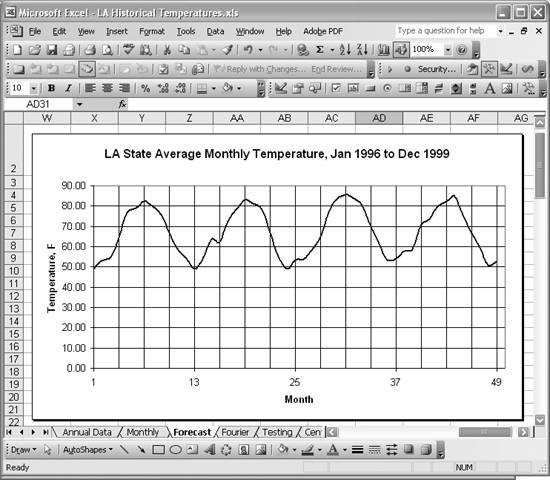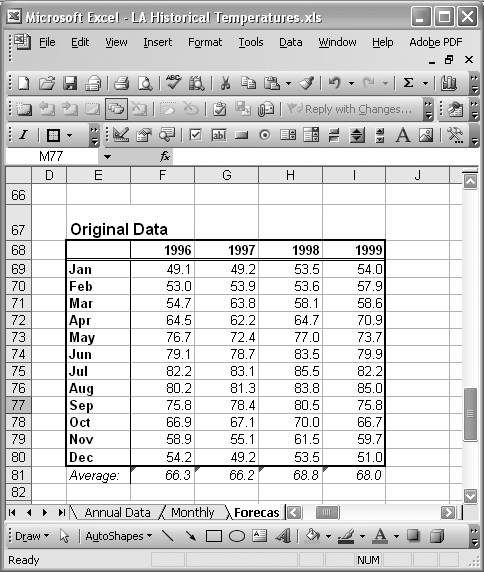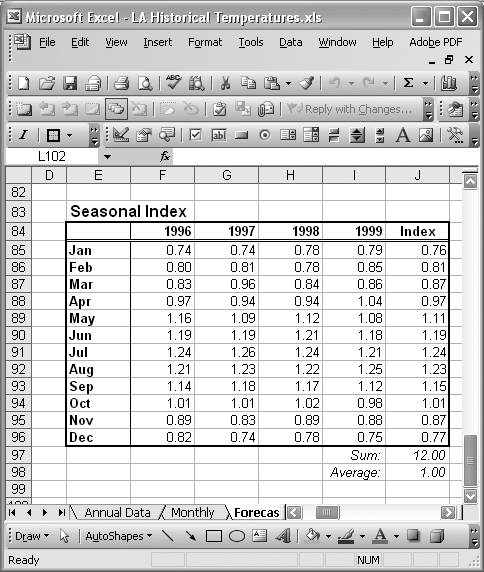# Estimating Seasonal Indices

Problem

You're working with a time series that shows some seasonal variation and you'd like to compute the seasonal indices prior to deseasonalizing the data.

Solution

You can compute seasonal indices using any of a number of methods. I'll show you how easy it is to compute such indices in Excel using the average-percentage method.

Discussion

Take a look at the time series shown in Figure 6-23.

Figure 6-23. Average monthly temperaturesThis time series consists of average monthly temperatures for the state of Louisiana from 1996 to 1999. The vertical gridlines correspond to three-month quarters, and it's clear there's an obvious seasonal variation in average temperatures. In Recipe 6.9, I'll show you how to forecast the average monthly temperatures for the year 2000 given this historical data. Part of that forecast analysis requires you to isolate the seasonal variation in temperatures. To do so, you must first compute the seasonal indices.

There are many standard methods for computing seasonal indices. The method I'll show you to illustrate how to use Excel for such calculations is the average-percentage method.

I'll use the series shown in Figure 6-23 as an example. To apply the average-percentage method, compute the annual average temperature for each year and then express each monthly temperature as a percentage of the average annual temperature for the corresponding year. Then you average these percentages for corresponding months over all years to arrive at the seasonal index for each month.

The data shown in Figure 6-23 was given as a series of temperature values over a range of months from 1 to 49, covering the years 1996 to 1999. To compute the seasonal indices, it's convenient to reorganize the data as shown in Figure 6-24.

Figure 6-24. Original data and averagesThe original data is now in a table with rows representing monthly values over years represented by columns. Now it's a simple matter of applying the AVERAGE worksheet formula to compute annual averages. The last row of the table in Figure 6-24 computes these averages using formulas of the form =AVERAGE(F69:F80).

The next step is to divide each monthly temperature value by the annual average for the corresponding year. Figure 6-25 shows the table I set up for this calculation.

Figure 6-25. Seasonal indicesThis table is similar in format to the one shown in Figure 6-24; however, each value in the table represents the ratio of monthly temperature to annual average for corresponding years. For example, the cell formula in cell F85 corresponding to January 1996 is =F69/F\$81, which is the temperature value for January 1996 from the table shown in Figure 6-24 divided by the annual average for the year 1996, from the last row of the table in Figure 6-24. The cell formulas are similar for the other months and years in the table in Figure 6-25.

The last column in Figure 6-25 contains the seasonal index for each month. The seasonal index is simply the average of the ratios for the corresponding month over all years. For example, the January seasonal index in cell J85 is computed using the formula =AVERAGE(F85:I85). The remaining indices are computed similarly.

The average of the seasonal indices for all months should come out to a value of 1. If it does not, then a suitable factor should be applied to each index so that the average does indeed work out to a value of 1. Cell J98 computes the average seasonal index as a check. As you can see, it comes out to a value of 1.00, so no adjustments are required.

The seasonal indices computed here confirm the observation made earlier that the temperatures are seasonally higher in the middle months of the year (over the second and third quarters of each year). You can see this by observing that the seasonal indices for the months of May through October are above the average index of 1, while the remaining indices are below this average.

You can decompose a time series such as the one discussed here to isolate the seasonal variation in a manner similar to the way in which we isolated the long-term trend in Recipe 6.6. Further, as you can also deseasonalize a time series. The next recipe shows you how.Excel Scientific and Engineering Cookbook (Cookbooks (OReilly))
ISBN: 0596008791
EAN: 2147483647
Year: N/A
Pages: 206
Authors: David M Bourg